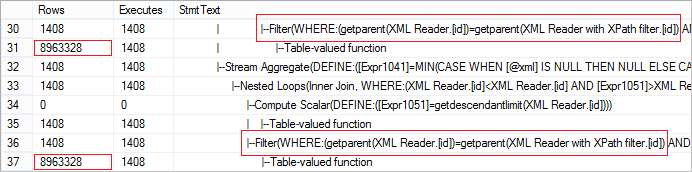# XML performance tips

Total: 6 Average: 3.5

Parsing data from XML using XQuery is a routine practice. In order to do this most effectively, little effort is required.

Suppose we need to parse data from the disk file with the following structure:

```<tables>
<table name="Accounting" schema="Production" object="Accounting">
<column name="Date" order="3" visible="1" />
<column name="DateFrom" order="5" visible="1" />
<column name="DateTo" order="6" visible="1" />
<column name="Description" order="4" visible="1" />
<column name="DocumentUID" order="1" visible="0" />
<column name="Number" order="2" visible="1" />
<column name="Warehouse" order="7" visible="1" />
</table>
</tables>```

Use BULK INSERT, if you need to read data from a file:

```SELECT BulkColumn
FROM OPENROWSET(BULK 'D:\data.xml', SINGLE_BLOB) x sample xml file```

A sample xml file is here.

However, bear in mind one particular thing… Try not to read the data directly:

```;WITH cte AS
(
SELECT x = CAST(BulkColumn AS XML)
FROM OPENROWSET(BULK 'D:\data.xml', SINGLE_BLOB) x
)
SELECT t.c.value('@name', 'VARCHAR(100)')
FROM cte
CROSS APPLY x.nodes('tables/table') t(c)```

Assign data to a variable. This way you can get a more efficient execution plan:

```DECLARE @xml XML
SELECT @xml = BulkColumn
FROM OPENROWSET(BULK 'D:\data.xml', SINGLE_BLOB) x

SELECT t.c.value('@name', 'VARCHAR(100)')
FROM @xml.nodes('tables/table') t(c)```

Compare the results:

```Table 'Worktable'. Scan count 0, logical reads 729, physical reads 0, read-ahead reads 0, lob logical reads 62655,...
SQL Server Execution Times:
CPU time = 1203 ms, elapsed time = 1214 ms.

SQL Server Execution Times:
CPU time = 16 ms, elapsed time = 4 ms.

SQL Server Execution Times:
CPU time = 0 ms, elapsed time = 3 ms.```

As you can see, the second option is substantially faster.

Another important feature of SQL Server when working with XQuery is that reading a parent element can result in poor performance. Consider the following example:

```SET STATISTICS PROFILE OFF

DECLARE @xml XML
SELECT @xml = BulkColumn
FROM OPENROWSET(BULK 'D:\data.xml', SINGLE_BLOB) x

SET STATISTICS PROFILE ON

SELECT
t.c.value('@name', 'SYSNAME')
, t.c.value('@order', 'INT')
, t.c.value('@visible', 'BIT')
, t.c.value('../@name', 'SYSNAME')
, t.c.value('../@schema', 'SYSNAME')
, t.c.value('../@object', 'SYSNAME')
FROM @xml.nodes('tables/table/*') t(c)```

Let’s look at the actual number of rows received from the operator. The value is abnormally large:The request can be easily optimized using CROSS APPLY:

```SELECT
t2.c2.value('@name', 'SYSNAME')
, t2.c2.value('@order', 'INT')
, t2.c2.value('@visible', 'BIT')
, t.c.value('@name', 'SYSNAME')
, t.c.value('@schema', 'SYSNAME')
, t.c.value('@object', 'SYSNAME')
FROM @xml.nodes('tables/table') t(c)
CROSS APPLY t.c.nodes('column') t2(c2)```

Let’s compare the execution time:

```(1408 row(s) affected)
SQL Server Execution Times:
CPU time = 10125 ms, elapsed time = 10135 ms.

(1408 row(s) affected)
SQL Server Execution Times:
CPU time = 78 ms, elapsed time = 156 ms.```

As you can see from the example, the request with CROSS APPLY works instantly.